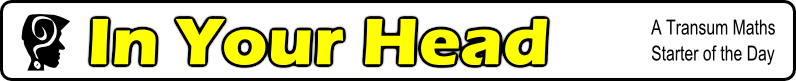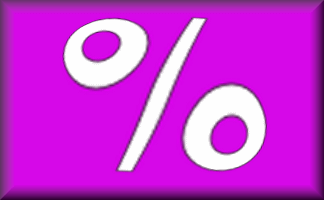#10% 50% 25% 15% £16 £22 £70 £5.40 £25.50 £80.40

Copy and complete this table in the back of your exercise book.
No calculator allowed!

Topics: Starter | Percentages

• Transum,
•
• This is a popular starter (as can be seen from the score below) and rightly so. It doesn't have the intrigue of some of our puzzle type starters but this starter provides pupils with practice of a very important life skill as well as a good mental arithmetic work out. A good understanding of percentages will help students develop strategies and short cuts for doing the calculations quickly. This is a starter that is well worth repeating, a different version is available here.
• Transum,
•
• HELP! How do you convert a fraction to a percentage? Do you multiply by 100 or do you multiply by 100% ? Textbooks differ and I have been having a conversation which is leaving me undecided. You can see the discussion here

How did you use this starter? Can you suggest how teachers could present or develop this resource? Do you have any comments? It is always useful to receive feedback and helps make this free resource even more useful for Maths teachers anywhere in the world.

Previous Day | This starter is for 29 September | Next Day

Teacher: If you are projecting this starter for your pupils to see note that the instructions directly underneath the table above are editable so you can change them to suit your circumstances.This activity is suitable for students of mathematics all around the world. Use the button below to change the currency symbol used to make it more relevant to your students. You may wish to choose an unfamiliar currency to extend your students' experience.% - This is the percent symbol.
Percent means 'out of 100'.

### Find 50%:

As 50 is half of 100, then 50% means half. To find 50% of a quantity you need to halve (or divide by two). So 50% of 6 is 3.

### Find 10%:

As 10 is one tenth of 100, then 10% means 'one tenth of'. To find 10% of a quantity you need to divide it by ten. So 10% of 800 is 80.

### Find 25%:

As 25 is one quarter of 100, then 25% means 'one quarter of'. To find 25% of a quantity you need to divide it by four. So 25% of 20 is 5.

Another way of finding 25% of a quantity is first finding 50% then dividing the result by 2.

### Find 33⅓%:

As 33⅓ is one third of 100, then 33⅓% means 'one third of'. To find 33⅓% of a quantity you need to divide it by three. So 33⅓% of 30 is 10.

### Find 1%:

As 1 is one hundredth of 100, then 1% means 'one hundredth of'. To find 1% of a quantity you need to divide it by 100. So 1% of 800 is 8.

### Find other percentages:

Other percentages can be found by combining some of the techniques mentioned above. Here are some examples:

• To find 75% of a quantity add together 50% and 25% of it.
• To find 20% of a quantity double 10% of it.
• To find 5% of a quantity halve 10% of it.
• To find 66⅔% of a quantity double 33⅓% of it.
• To find 90% of a quantity subtract 10% from it.
• To find 80% of a quantity subtract 20% from it.
• To find 2% of a quantity double 1% of it.
• To find 52% of a quantity add 50% to 2% of it.

If you need to use a calculator to check your working. See Calculator Workout skill 3.

### Commute

Did you know that if you are struggling to mentally work out 24% of 50 you can switch the numbers round and work out 50% of 24 instead. Finding 50% is very easy isn’t it? You will get the same answer.

Finding a percentage of a quantity is an example of a commutative calculation. Not all operations are commutative. Subtraction certainly isn’t as 10 minus one is not the same as one minus ten.

You can use this trick to improve your ability to do this type of calculation quickly if you find the switch makes it easier.

Practise with 12% of 50, 4% of 25 and 75% of 10.

Note to teacher: Doing this activity once with a class helps students develop strategies. It is only when they do this activity a second time that they will have the opportunity to practise those strategies. That is when the learning is consolidated. Click the link above to find another version of this starter or you can manually change the values in the table above.

Your access to the majority of the Transum resources continues to be free but you can help support the continued growth of the website by doing your Amazon shopping using the links on this page. Below is an Amazon link. As an Amazon Associate I earn a small amount from qualifying purchases which helps pay for the upkeep of this website.

Educational Technology on AmazonTeacher, do your students have access to computers such as tablets, iPads or Laptops?  This page was really designed for projection on a whiteboard but if you really want the students to have access to it here is a concise URL for a version of this page without the comments: Transum.org/go/?Start=September29 However it would be better to assign one of the student interactive activities below.Here is the URL which will take them to a student version of this activity.

Transum.org/go/?Num=60## After Thought

Which is the best bargain?

 Game ConsolesSALE20% off RecommendedRetail Price Game ConsolesSALE20% of RecommendedRetail Price

## Curriculum Reference

See the National Curriculum page for links to related online activities and resources.For All: